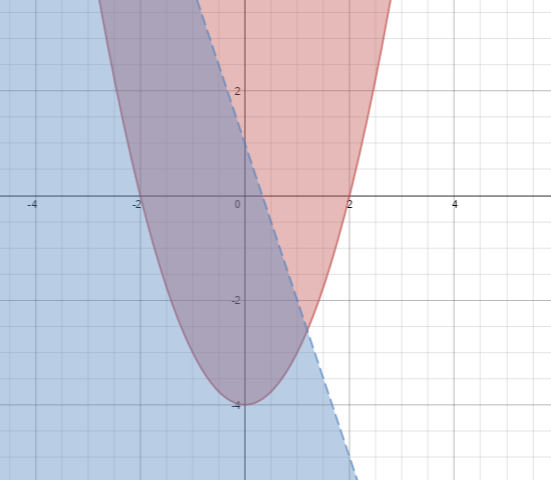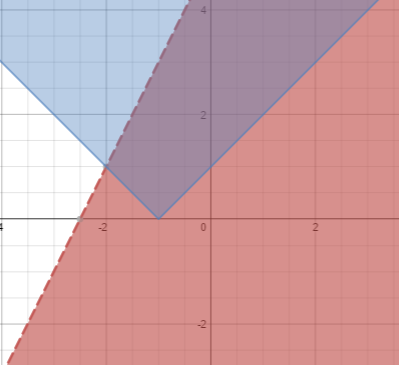### Home > INT3 > Chapter 6 > Lesson 6.1.3 > Problem6-29

6-29.

Graph each system and shade the solution region.

1. $y\ge x^2-4$
$y<−3x+1$

Graph each function and decide if the curves should be dashed or solid. Then shade the appropriate region.1. $y<2x+5$
$y\ge\left|x+1\right|$

Graph each function and decide if the lines should be dashed or solid. Then shade the appropriate region.